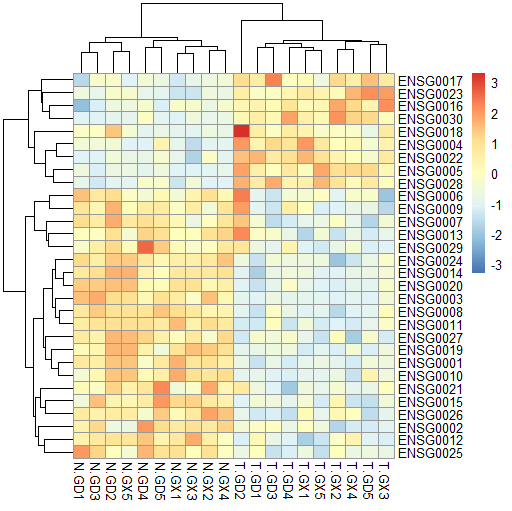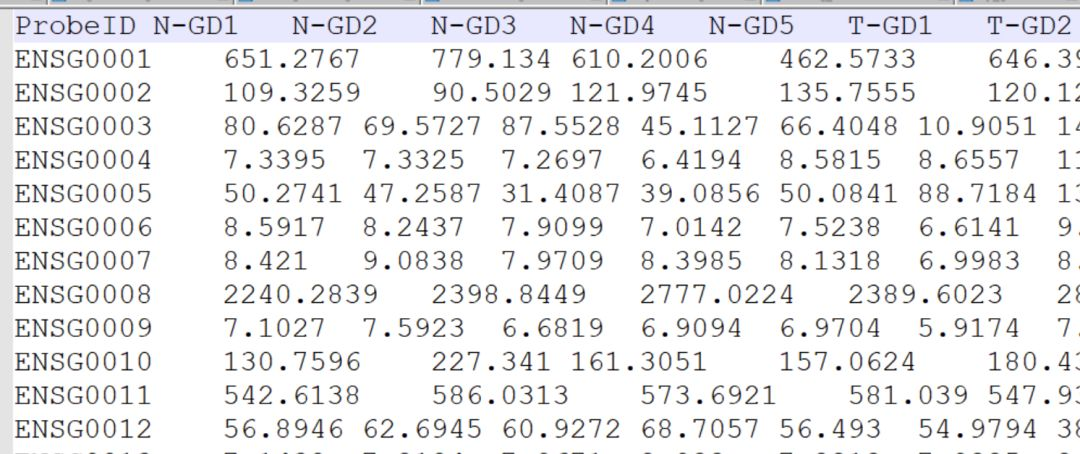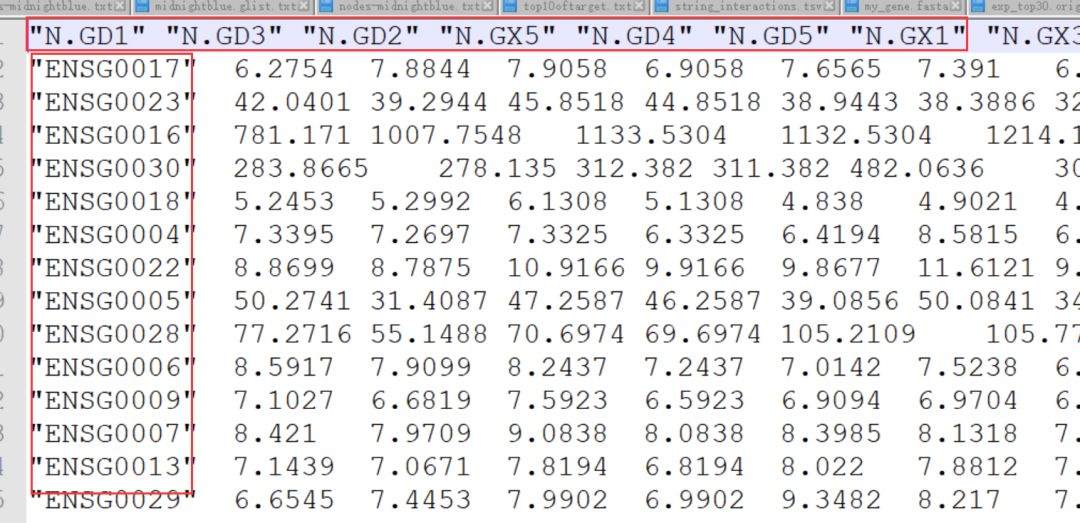# 如何按照热图中的顺序输出表达量文件#########分割线##########

library(pheatmap)

result <- pheatmap(exp,scale="row") # 保存热图的绘图关键数据## 从热图中提取样本顺序的编号和样本名

col_oder=result\$tree_col\$order # 保存热图列顺序（序号）

row_oder=result\$tree_row\$order # 保存热图行顺序（序号）

cn_new <- colnames(exp)[col_oder] # 保存热图的列名

rn_new <- rownames(exp)[row_oder] # 保存热图的行名

## 生成两个新的与原来的表达量总表长宽相同的数据框（暂时都填写0）

new_exp <- matrix(rep(0,ncol(exp)*nrow(exp)),nrow = nrow(exp),ncol = ncol(exp))

out <- matrix(rep(0,ncol(exp)*nrow(exp)),nrow = nrow(exp),ncol = ncol(exp))

## 将表达量数据读入数据框new_exp,行顺序已经按照热图的顺序重排

for (i in 1:ncol(exp)){

new_exp[,i]=exp[,i][row_oder]

}

## 将表达量数据读入数据框out,列顺序已经进一步按照热图的顺序重排

for (i in 1:ncol(exp)){

out[,i]= new_exp[,col_oder[ i ]]

}

# 将热图的行名和列名导入到排序后的表达量总表中

rownames(out)=rn_new

colnames(out)=cn_new

write.table(out,"hp_exp.txt",sep="t") #输出重排后的表达量表

############################返回搜狐，查看更多